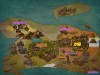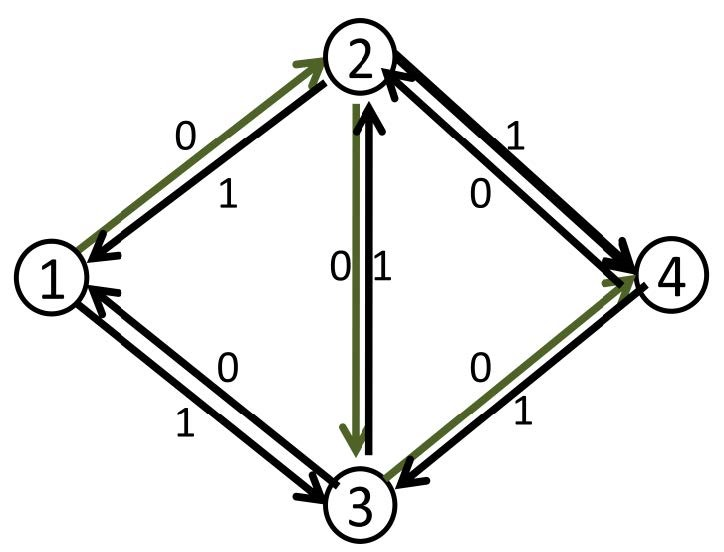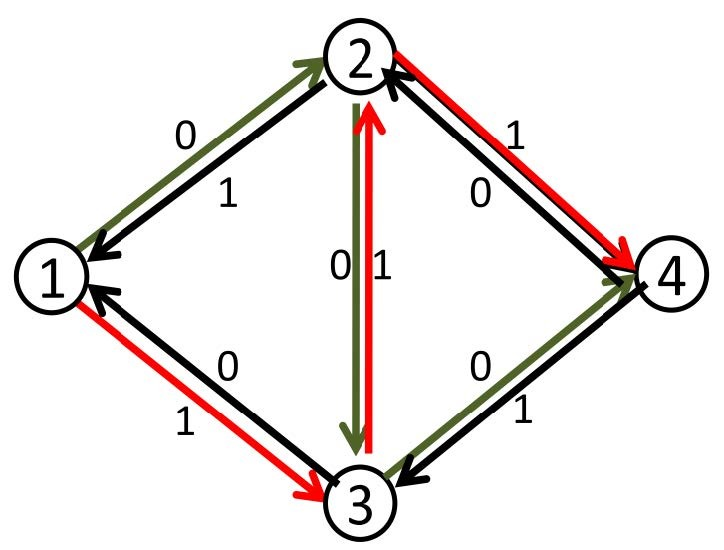星星灰暗着。星星灰暗着。

玄学算法/精彩DS总结 Tascho/Memairos

posted on 2018-12-07 14:14:25 | under Summary |

$1.Link-Cut\ Tree(including\ Splay)$

$Attention:FlashHu$ 博客中的 $LCT$ 图片仅为示意图，不保证正确性。
$LCT$ 是一种奇妙的数据结构。尽管其理论复杂度是 $O(m\log n)$ ，但其优秀的常数使得其可以媲美 $O(m\log^2 n)$ 甚至 $O(m\log^3 n)$ 。

$LCT$ 有一些优美的性质：

$rotate$

$Splay$ 是依靠旋转来维持平衡的。

$link$

$link$ 后不能 $pushup(y)!$ 尽管看似 $pushup(y)$ 是无意义的行为，但其会导致错误。原因不明。

$cut$

$1.findroot(y)!=x$ ；
$2.fa[x]!=y$ ；
$3.son[x]$ 有值。

$split$

$makeroot(x),access(y),splay(y)$ 即可。此时 $y$ 上的信息就是整条链的信息。

$pushup,pushdown$

$pushup$ 的主要作用是将链上信息上传。
$pushdown$ 的主要作用是执行翻转标记，同时将链修改信息下传。

$subtree-access$

$access$ 会直接改变虚实儿子的关系，所以维护信息的时候要改一下。

$2.Suffix\ Automaton$

$right/end-pos\ set$

$endpos/right$ 集合的包含关系实际上形成了 $parent$ 树。

$3.Network\ Flow$

$Edmonds-Karp$

$\cdots\cdots$ 当然，在这之前，还是要讲一下朴素的最大流算法。（图片出自https://www.cnblogs.com/ZJUT-jiangnan/p/3632525.html）

$Capacity\ Scaling$ 玄学

$Capacity\ Scaling$ 指的是，在每次 $Dinic$ 算法的执行过程前，先设定一个权值 $val$ ，然后在整个 $Dinic$ 算法的执行过程中，只有残量 $\ge val$ 的边才会被计算。譬如 $BFS$ 分层的的时候找的只能是 $cap\ge val$ 的边，增广时亦然。

$DAG\ Minimum\ Path\ Cover$

$DAG$ 上的最小路径覆盖是可以使用多项式算法解决的。

$DAG\ Minimum\ Chain\ Cover$

$DAG$ 最小链覆盖的求解就是先传递闭包，再进行最小路径覆盖。感性理解的话就是通过传递闭包让原来要通过多条边才能到达的两个点直接有一条路径连接，就不会造成与其它路径相交的问题。因此这样的路径覆盖就是原图的最小链覆盖。

$Hall\ Theorem$

$Hall$ 定理指的是，一个二分图左右分别有 $m\le n$ 个点，那么若它存在完备匹配，当且仅当左边任意 $k(k\in[1,m])$ 个点至少与右边 $k$ 个点相邻。